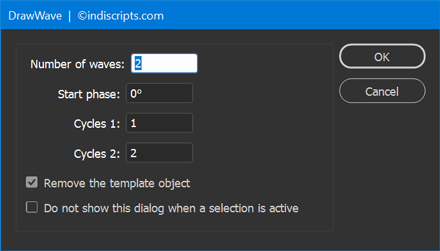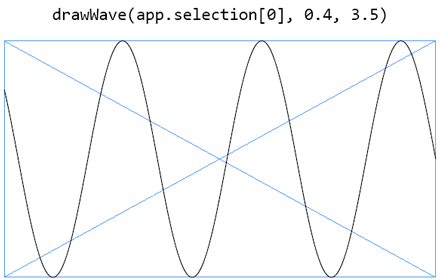Update 21-Mar-2021. — This article and the attached script was originally posted in the “Tips” section due to its technical flavor. However, as the code has evolved and now provides a dialog box that allows the user to easily enter custom settings, the material is now delivered as a standalone script (DrawWave.jsx) that you can just install and use without having to sink into its mathematical arcana…At its most superficial level, the routine DrawWave.js brings a snippet that takes a `PageItem` as a canvas and creates a sine wave fitting the target area. This curve is not made of straight-line samples which wouldn't scale smoothly. Instead, we approximate the sine function using a cubic Bézier curve that renders each quarter of the period, `0..π/2`, `π/2..π`, `π..3π/2`, etc.

The `drawWave()` function supports two additional arguments expressing the working interval. Keep the default parameters 0 and 1—that is, `drawWave(canvas,0,1)`—to generate a full `[0,2π]` sine wave portion with no phase:But you can also specify a starting point “in 2π units” (`0.25` for `π/2`, `0.5` for `π`, etc.) and the final location as well (`1` for `2π`, `1.5` for `3π`, `2` for `4π`…). Thus any possible sine curve can be described:## Looking Closer

In fact, no Bezier spline can exactly fit each point of an ideal sine wave. One can just provide control points that interpolate slope and curvature in [0,π/2] with minimal error.Then, given an optimal spline for the first quarter `0..π/2` everything else follows using the symmetry and periodicity properties of the sine function. In this project, however, my goal was to support arbitrary phases within `[0,2π[` and arbitrary lengths beyond `2π`, including non-integer multiples of `π/2`. This approach leads to interesting topics:
• Preventing cascading floating-point errors during calculations,
• Translating orthonormal (x,y) coordinates into the specific bounding box system that takes for origin the top-left anchor point and for unit basis the left-to-right and top-to-bottom vectors,
• Locating a point on a cubic Bézier curve, that is, finding the parameter `t` for which `(x(t),y(t))` meets some requirements—in the present case, `x(t)==0` for the starting point `A` and `x(t)==1` for the endpoint `B`,
• Splitting the Bezier curve in `A`, then in `B`, without invoking tricky Pathfinder-based operations.Give a look at the source code to study how these questions have been addressed. Special mention should be made of A Primer on Bézier Curves (by Mike “Pomax” Kamermans), probably the greatest resource ever designed for Bézier fans and programmers. Its “Splitting curves using matrices” section contains all the stuff behind my splitting routine.

## Box to Ruler Conversion

As it may happen from time to time on this website ;-) a hidden gem is buried in the script. The function `boxToRulerMatrix()` takes a `PageItem` and returns a matrix that maps the bounding box space into the current ruler system whatever the units in use, the zero point, and so on.

This is a powerful helper for those who need to easily generate `PathPoint` coordinates, or entire paths, from abstract data that only describe the inner geometry of a spline item.

Note that `boxToRulerMatrix()` invokes a function, `parentSpread`, which finds the parent `Spread` of some object. Here is the code of the converter (I use it in many other scripts):

```var parentSpread = function F(/*DOM*/o)
//----------------------------------
// Return the parent spread of an object, if any
{
var p = o && o.parent;
if( (!p) || (p instanceof Document) ) return null;
p : F(p);
};

var boxToRulerMatrix = function(/*PageItem*/o)
// -------------------------------------
// Given a page item, return a matrix that maps its
// box space (0..1, 0..1) into the current ruler system
{
const CS_BOARD = +CoordinateSpaces.PASTEBOARD_COORDINATES,
CS_INNER = +CoordinateSpaces.INNER_COORDINATES,
BB_GEO = +BoundingBoxLimits.GEOMETRIC_PATH_BOUNDS,
AP_TOP_LEFT = +AnchorPoint.TOP_LEFT_ANCHOR;

ref = spd && spd.pages,
bo, bs, ro, rs, mx;

if( !ref ) return 0;

// Box origin --> CS_INNER (trans)
// ---
bo = o.resolve([[0,0],BB_GEO,CS_INNER], CS_INNER);

// Box (u,v) --> CS_INNER (scaling)
// ---
bs = o.resolve([[1,1],BB_GEO,CS_INNER], CS_INNER);
bs -= bo;
bs -= bo;

// Ruler origin --> CS_BOARD (trans)
// ---
ro = ref.resolve([[0,0],AP_TOP_LEFT], CS_BOARD, true);

// Ruler (u,v) --> CS_BOARD (scaling)
// ---
rs = ref.resolve([[1,1],AP_TOP_LEFT], CS_BOARD, true);
rs -= ro;
rs -= ro;